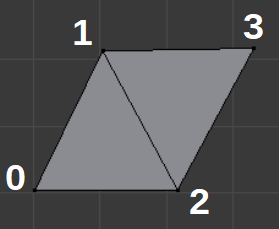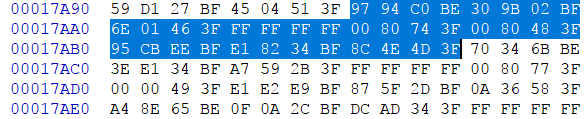# Reverse Engineering NFSU2 Part 2 - Finding Vertices

Mesh data is usually made of four things - vertices, normals, texture coordinates, and indices. These things describe the shape and appearance of triangles, which connect together to make the shape of your favorite game characters, worlds, and any other 3d objects.

Vertices are the points that make up the triangles (each triangle needs 3, one for each corner). Each vertex has a cartesian coordinate describing its position in space. Vertex positions are written in the form (x, y, z), and you will usually see them stored as triplets of floats.

Normals are direction vectors. At every vertex, there is a normal describing which way the surface is facing, to be used in lighting calculations. They also come in triplets (nx, ny, nz). If you drew a line from (0, 0, 0) to (nx, ny, nz), the direction of that line is the direction of the normal. Normals get their name because they are “normalized,” meaning they have a length of one. This is mathematically described by the distance formula:

`nx^2 + ny^2 + nz^2 = 1^2`

Texture coordinates are 2D. Basically, plain triangles look plain, so if you want to make them look like a surface with a texture (for example, the texture of bricks, or of pavement) you overlay an image over them. Games map points on the image to points on their 3d triangles with 2d coordinates in the form (u, v). U=0 usually means a vertex is on the far left of an image and u=1 means the right. v=0 is the bottom of the image and v=1 is the top. Sometimes the convention for v is reversed though, meaning the top of the screen is 0 and the bottom is 1.

Lastly there are indices. Every triangle needs 3 vertices. The triangles in a mesh typically share a lot of vertices, however. A simple mesh with two connected triangles will only need 4 unique vertices.To save memory (and improve speed, since the gpu doesn’t have as much repeated data to sift through) you store the vertices, normals, and texture coordinates in arrays, and then describe each triangle with indexes to the array. Indices for the mesh above would be listed like this in memory: 0, 2, 1; 2, 3, 1. The first three indices say which vertices make the first triangle, the second three are for the second triangle. A single index usually refers to the vertex, normal and tex coord at a point, though it is possible to have different indices for each.

Vertex, normal, and tex coord data can be interleaved (x, y, z, nx, ny, nz, u, v), (x, y, z, nx, ny, nz, u, v), (x, y, z, nx, ny, nz, u, v)…, or it can be in separate lists (x, y, z), (x, y, z), (x,y,z)… and then (nx, ny, nz), (nx, ny, nz)…you get the point. It’s up to the programmers.

## Finding Stuff:

So if you have a 10MB file that you know little about, how do you find this data? With code of course. I wrote a program which pretends the entire file is floating point numbers. It then looks for 3 float sequences where each number in the sequence is in the range [0.001, 10000] and [-10000, -.0001]. Game designers can set the scale in their game however they want. One unit could mean a centimeter, a meter, a mile, or anything, but ultimately people avoid working with really huge or really small values when they can, so this range is a good place to start looking. This method has flaws of course. You will miss some values (zeros in particular), and you will also have many false positives (this method can’t distinguish a vertex from a normal, a color, or any sequence of 3 bytes that just happens to also code for a float in this range). When you see the big clusters of potential vertices in a file, though, you know where to look for these things.

Perhaps a better way is to look for normals. This is the same bit of code, only it checks that the 3 values it’s looking code for a vector of length 1.0.

Running the normal finder on a Geometry.bin file for the Audi in NFSU2, and grabbing a random spot in it, I see this:

`(-0.229692220688, 0.706561923027, 0.669336736202) @ 17A74(-0.376133650541, -0.510180473328, 0.7734593153) @ 17A98(-0.229692220688, -0.706561923027, 0.669336736202) @ 17ABC(-0.224176943302, -0.67202848196, 0.70577788353) @ 17AE0`

These possible normals are all 0x24 (36) bytes apart. This is space for 9 floats (4 bytes/float), 3 of which are supposed normals. Could the 6 bytes between normals be vertex and tex coord data? Here is the data at 0x17A98 in a hex editor:As floats the bytes are:

`(-0.376, -0.510, 0.773, NaN, .955, .783, -1.87, -.705, .802)`

The first three are the normal found by the program. Next is a NaN, or something other than a float, actually 0xFFFFFFFF. Not sure what to do with this. The three bytes following could be a position, but that leaves an awkward two floats on the end, one of which is negative, so the end bytes probably aren’t a texture coordinate. A more likely guess is that the two numbers after the NaN, both of which fall between 0 and 1, are the texture coordinate. The last three bytes are a position.

Looking at other 36 byte snippets confirms the pattern. If you go to a spot where normals are first found after a gap in the file, you can see that the position floats begin the pattern. So the mesh data is in this format:

`(px, py, pz, nx, ny, nz, 0xFFFFFFFF, u, v)`

Cool. We’ve got mesh data. Now to do something with it…